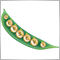# how to calculate MA on RSI?219

I want to calculate MA of RSI .

SO far I could come this far with this code:

```double rsi[];

for(int i=0 ; i<=100 ; i++)
{
rsi[i]=iRSI(Symbol(),0,14,PRICE_OPEN,i);
}

ArraySetAsSeries(rsi,true);
double ma=iMAOnArray(rsi,0,5,0,MODE_EMA,1);
Print("MA value ",ma);```

but when I compile the above EA I see this message in Experts tab of MT4:

2014.11.01 14:28:07.723    array out of range in 'maOnRSI.mq4' (50,10)

how can I solve this? what am I doing wrong?

ThanksModerator
32147

You are declaring a dynamic array

`double rsi[];`

but you don't size it, you have to use ArrayResize219

GumRai:

You are declaring a dynamic array

but you don't size it, you have to use ArrayResize

OK , I went one step forward, but there is still a problem:

I am using the following code, but the problem is I want to calculate MA -> period=5, MA method=Exponential, shift=0,      on RSI with (period=14, Apply to=Open).

MA of RSI for bar 1 in the indicators that I have installed on the chart is = 66.4862 but the following code says it is :

2014.11.01 15:09:07.336    maOnRSI GBPJPY,M15: MA of RSI for bar= 1 = 0.1348944125100005

what am I doing wrong now?

```void OnTimer()
{
//---
double RSIBuffer[];
double MAofRSIBuffer[];
ArrayResize(RSIBuffer,Bars+1);
ArrayResize(MAofRSIBuffer,Bars+1);
for(int i=0 ; i<=Bars ; i++)
{
RSIBuffer[i]=NormalizeDouble( iRSI(NULL,0,14,PRICE_OPEN,i) ,4);

}

for(int i=0; i <= 4; i++)
{
MAofRSIBuffer[i]=iMAOnArray(RSIBuffer,0,5,0,MODE_EMA,i);
Print ("IMAOnArray for ",i," = ",NormalizeDouble (iMAOnArray(RSIBuffer,0,5,0,MODE_EMA,i) ,4));
}
Print ("rsi = ",RSIBuffer);
Print ("MA of RSI for bar= ", 1," = ",MAofRSIBuffer);

}```219

sam2014:

OK , I went one step forward, but there is still a problem:

I am using the following code, but the problem is I want to calculate MA -> period=5, MA method=Exponential, shift=0,      on RSI with (period=14, Apply to=Open).

MA of RSI for bar 1 in the indicators that I have installed on the chart is = 66.4862 but the following code says it is :

2014.11.01 15:09:07.336    maOnRSI GBPJPY,M15: MA of RSI for bar= 1 = 0.1348944125100005

what am I doing wrong now?8033

sam2014:

OK , I went one step forward, but there is still a problem:

I am using the following code, but the problem is I want to calculate MA -> period=5, MA method=Exponential, shift=0,      on RSI with (period=14, Apply to=Open).

MA of RSI for bar 1 in the indicators that I have installed on the chart is = 66.4862 but the following code says it is :

2014.11.01 15:09:07.336    maOnRSI GBPJPY,M15: MA of RSI for bar= 1 = 0.1348944125100005

what am I doing wrong now?

Try this

```void OnTimer()
{
//---
int i,j;
int signal=5;
double RSIBuffer[];
double MAofRSIBuffer[];
ArrayResize(RSIBuffer,signal*2);
ArrayResize(MAofRSIBuffer,signal+2);
for(i=0 ; i<signal*2 ; i++)
{
RSIBuffer[i] = iRSI(NULL,0,14,PRICE_OPEN,i);

}

ArraySetAsSeries(RSIBuffer,true);

for(i=0; i <signal+2; i++)
{
MAofRSIBuffer[i]=iMAOnArray(RSIBuffer,0,siganl,0,MODE_EMA,i);
Print ("IMAOnArray for ",i," = ",iMAOnArray(RSIBuffer,0,signal,0,MODE_EMA,i));
}
Print ("rsi = ",RSIBuffer);
Print ("MA of RSI for bar= ", 1," = ",MAofRSIBuffer);

}```25838

 pipPod: Try this With`ArraySetAsSeries(rsi,true);`Before filling in the values.8033

WHRoeder:
 pipPod: Try this WithBefore filling in the values.
Fixed.